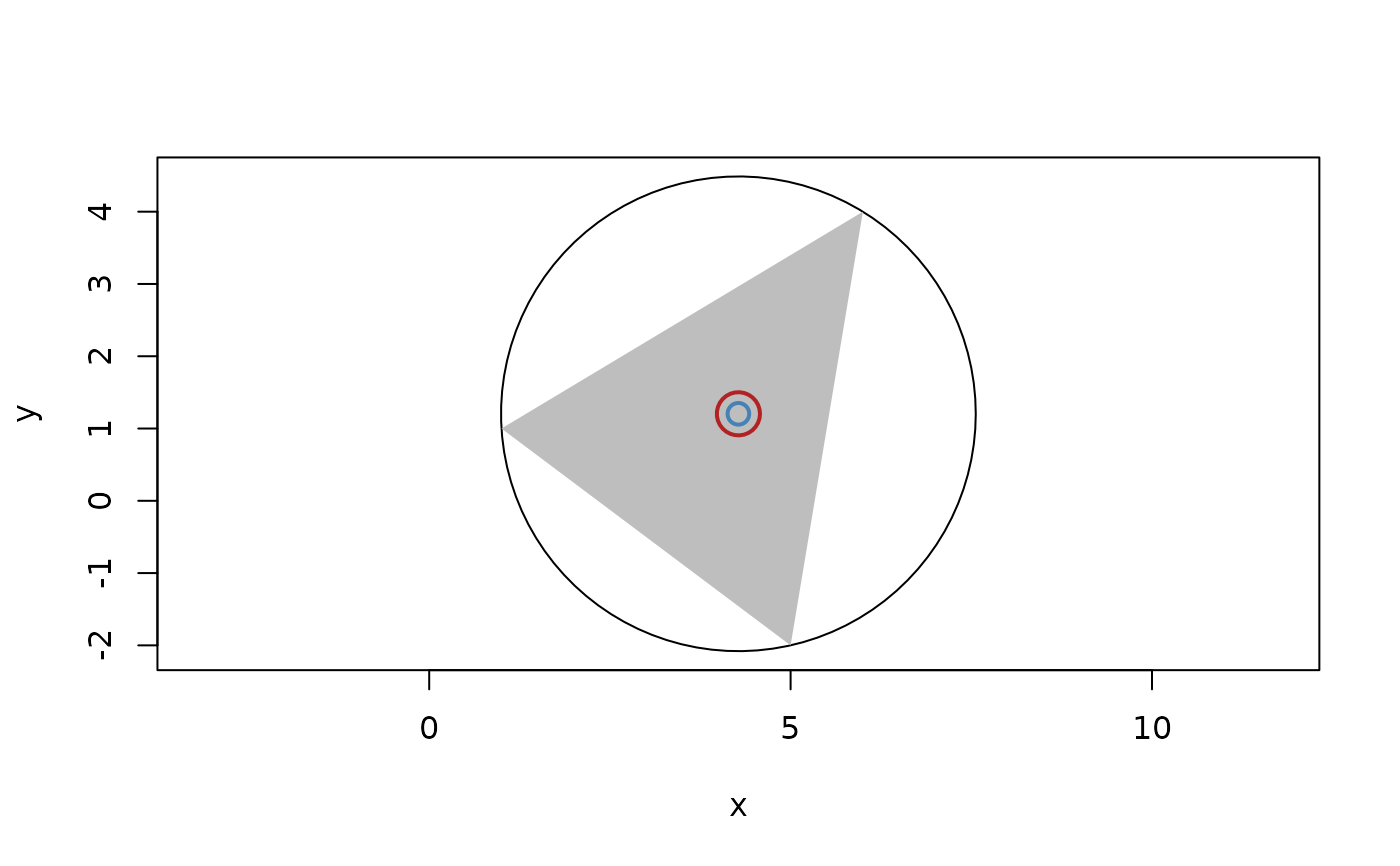The circumcenter is the center of the circle of sphere passing through the vertices of a geometry (or the provided points).

## Usage

circumcenter(...)

## Arguments

...

geometry vectors. Either a triangle or tetrahedron vector, or 2, 3, or 4 point vectors. If 4 are provided they must be in 3D

## Value

A points vector

Other Locations: barycenter(), bisector(), centroid(), equidistant_line(), point(), radical(), weighted_point()

## Examples

# Circumcenter of triangle
t <- triangle(point(1, 1), point(5, -2), point(6, 4))
circumcenter(t)
#> <2D points >
#>  <x:4.28, y:1.2>

# Same as providing the points directly
circumcenter(point(1, 1), point(5, -2), point(6, 4))
#> <2D points >
#>  <x:4.28, y:1.2>

# Same as getting the center of a circle constructed from the points
circ <- circle(point(1, 1), point(5, -2), point(6, 4))
vert(circ)
#> <2D points >
#>  <x:4.28, y:1.2>

plot(circ)
euclid_plot(t, col = "grey", border = NA)
euclid_plot(circumcenter(t), cex = 3, col = "firebrick", lwd = 2)
euclid_plot(vert(circ), cex = 1.5, col = "steelblue", lwd = 2)